# The Unscented Kalman Filter¶

In :
%matplotlib inline

In :
#format the book
import book_format
book_format.set_style()

Out:

In the last chapter we discussed the difficulties that nonlinear systems pose. This nonlinearity can appear in two places. It can be in our measurements, such as a radar that is measuring the slant range to an object. Slant range requires you to take a square root to compute the x,y coordinates:

$$x=\sqrt{\text{slant}^2 - \text{altitude}^2}$$

The nonlinearity can also occur in the process model - we may be tracking a ball traveling through the air, where the effects of air drag lead to nonlinear behavior. The standard Kalman filter performs poorly or not at all with these sorts of problems.

In the last chapter I showed you a plot like this. I have altered the equation somewhat to emphasize the effects of nonlinearity.

In :
from kf_book.book_plots import set_figsize, figsize
import matplotlib.pyplot as plt
from kf_book.nonlinear_plots import plot_nonlinear_func
from numpy.random import normal
import numpy as np

# create 500,000 samples with mean 0, std 1
gaussian = (0., 1.)
data = normal(loc=gaussian, scale=gaussian, size=500000)

def f(x):
return (np.cos(4*(x/2 + 0.7))) - 1.3*x

plot_nonlinear_func(data, f)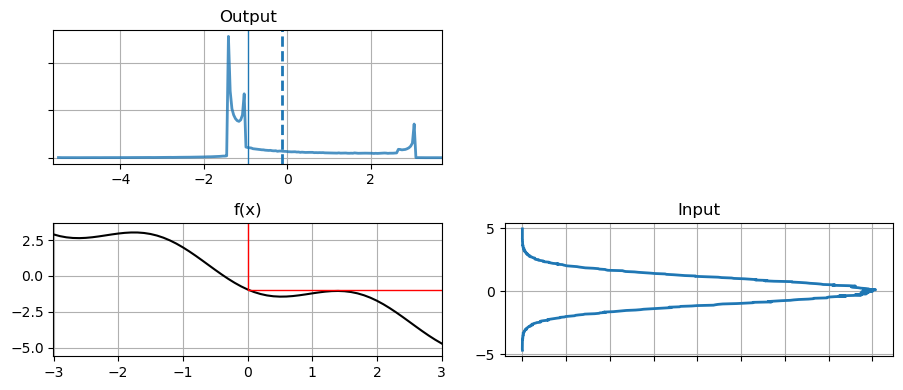I generated this by taking 500,000 samples from the input, passing it through the nonlinear transform, and building a histogram of the result. We call these points sigma points. From the output histogram we can compute a mean and standard deviation which would give us an updated, albeit approximated Gaussian.

Let me show you a scatter plot of the data before and after being passed through f(x).

In :
N = 30000
plt.subplot(121)
plt.scatter(data[:N], range(N), alpha=.2, s=1)
plt.title('Input')
plt.subplot(122)
plt.title('Output')
plt.scatter(f(data[:N]), range(N), alpha=.2, s=1);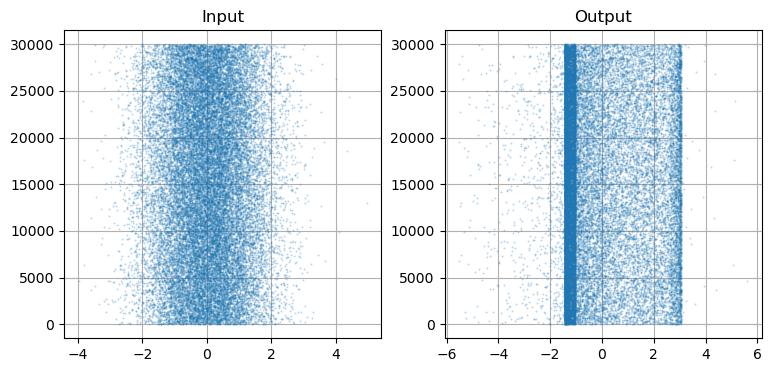The data itself appears to be Gaussian, which it is. By that I mean it looks like white noise scattered around the mean zero. In contrast g(data) has a defined structure. There are two bands, with a significant number of points in between. On the outside of the bands there are scattered points, but with many more on the negative side.

It has perhaps occurred to you that this sampling process constitutes a solution to our problem. Suppose for every update we generated 500,000 points, passed them through the function, and then computed the mean and variance of the result. This is called a Monte Carlo approach, and it used by some Kalman filter designs, such as the Ensemble filter and particle filter. Sampling requires no specialized knowledge, and does not require a closed form solution. No matter how nonlinear or poorly behaved the function is, as long as we sample with enough sigma points we will build an accurate output distribution.

"Enough points" is the rub. The graph above was created with 500,000 sigma points, and the output is still not smooth. What's worse, this is only for 1 dimension. The number of points required increases by the power of the number of dimensions. If you only needed 500 points for 1 dimension, you'd need 500 squared, or 250,000 points for two dimensions, 500 cubed, or 125,000,000 points for three dimensions, and so on. So while this approach does work, it is very computationally expensive. Ensemble filters and particle filters use clever techniques to significantly reduce this dimensionality, but the computational burdens are still very large. The unscented Kalman filter uses sigma points but drastically reduces the amount of computation by using a deterministic method to choose the points.

## Sigma Points - Sampling from a Distribution¶

Let's look at the problem in terms of a 2D covariance ellipse. I choose 2D merely because it is easy to plot; this extends to any number of dimensions. Assuming some arbitrary nonlinear function, we will take random points from the first covariance ellipse, pass them through the nonlinear function, and plot their new position. Then we can compute the mean and covariance of the transformed points, and use that as our estimate of the mean and probability distribution.

In :
import kf_book.ukf_internal as ukf_internal
ukf_internal.show_2d_transform()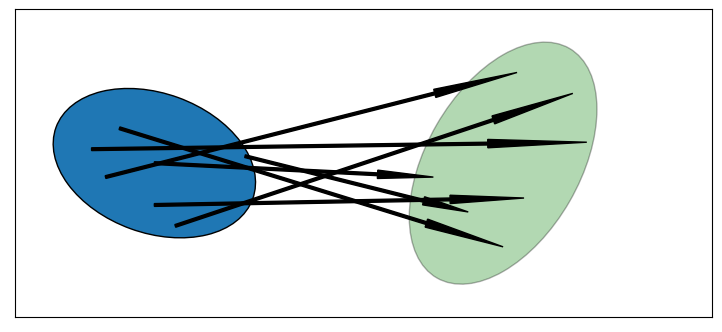On the left we show an ellipse depicting the $1\sigma$ distribution of two state variables. The arrows show how several randomly sampled points might be transformed by some arbitrary nonlinear function to a new distribution. The ellipse on the right is drawn semi-transparently to indicate that it is an estimate of the mean and variance of this collection of points.

Let's write a function which passes 10,000 points randomly drawn from the Gaussian

$$\mu = \begin{bmatrix}0\\0\end{bmatrix}, \Sigma=\begin{bmatrix}32&15\\15&40\end{bmatrix}$$

through the nonlinear system:

\begin{cases}\begin{aligned}\bar x&=x+y\\ \bar y&= 0.1x^2 + y^2\end{aligned} \end{cases}

In :
import numpy as np
from numpy.random import multivariate_normal
from kf_book.nonlinear_plots import plot_monte_carlo_mean

def f_nonlinear_xy(x, y):
return np.array([x + y, .1*x**2 + y*y])

mean = (0., 0.)
p = np.array([[32., 15.], [15., 40.]])
# Compute linearized mean
mean_fx = f_nonlinear_xy(*mean)

#generate random points
xs, ys = multivariate_normal(mean=mean, cov=p, size=10000).T
plot_monte_carlo_mean(xs, ys, f_nonlinear_xy, mean_fx, 'Linearized Mean');

Difference in mean x=-0.055, y=43.117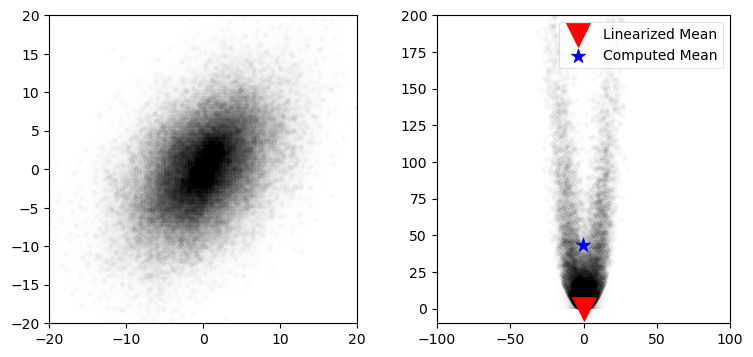This plot shows the strong nonlinearity that occurs with this function, and the large error that would result if we linearized in the way of the Extended Kalman filter (we will be learning this in the next chapter).

## A Quick Example¶

I'm soon going to proceed into the math the Unscented Kalman Filter (UKF) uses to choose sigma points and then perform the computation. But let's just start with an example so you can see the destination, so to speak.

We will learn that the UKF can use many different algorithms to generate the sigma points. Several algorithms are provided by FilterPy. Here's one possibility:

In :
from filterpy.kalman import JulierSigmaPoints

sigmas = JulierSigmaPoints(n=2, kappa=1)


This will become clearer later, but the object will generate weighted sigma points for any given mean and covariance. Let's just look at an example, where the point's size indicate how much it is weighted:

In :
from kf_book.ukf_internal import plot_sigmas
plot_sigmas(sigmas, x=[3, 17], cov=[[1, .5], [.5, 3]])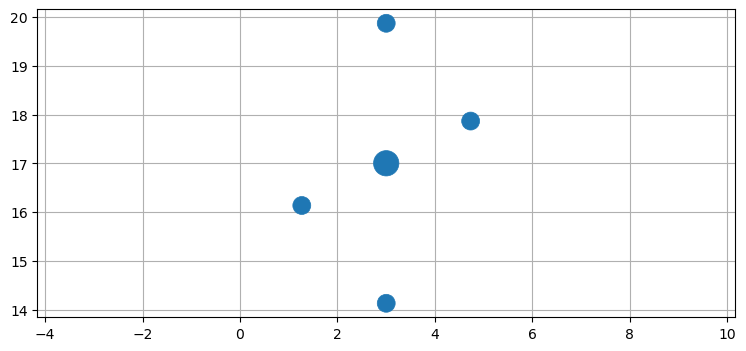You can see we have 5 points centered around the mean (3, 17) in an odd pattern. It may seem absurd that this will do as well or better than 500,000 randomly generated points, but it will!

Okay, now let's implement the filter. We will implement a standard linear filter in 1D; we aren't quite ready to tackle a nonlinear filter yet. The design of the filter is not much different than what we have learned so far, with one difference. The KalmanFilter class uses the matrix $\mathbf F$ to compute the state transition function. Matrices mean linear algrebra, which work for linear problems, but not nonlinear ones. So, instead of a matrix we provide a function, just like we did above. The KalmanFilter class uses another matrix $\mathbf H$ to implement the measurement function, which converts a state into the equivalent measurement. Again, a matrix implies linearity, so instead of a matrix we provide a function. Perhaps it is clear why $\mathbf H$ is called the 'measurement function'; for the linear Kalman filter it is a matrix, but that is just a fast way to compute a function that happens to be linear.

Without further ado, here are the state transistion function and measurement function for a 1D tracking problem, where the state is $\mathbf x = [x \, \, \dot x]^ \mathsf T$:

In :
def fx(x, dt):
xout = np.empty_like(x)
xout = x * dt + x
xout = x
return xout

def hx(x):
return x[:1] # return position [x]


Let's be clear, this is a linear example. There is no need to use a UKF for a linear problem, but I'm starting with the simplest possible example. But notice how I wrote fx() to compute $\mathbf{\bar x}$ as a set of equations instead of a matrix multiplication. This is to illustrate that I could implement any arbitrary nonlinear function here; we are not constrained to linear equations.

The rest of the design is the same. Design $\mathbf P$, $\mathbf R$, and $\mathbf Q$. You know how to do that, so let's just finish up the filter and run it.

In :
from numpy.random import randn
from filterpy.kalman import UnscentedKalmanFilter
from filterpy.common import Q_discrete_white_noise

ukf = UnscentedKalmanFilter(dim_x=2, dim_z=1, dt=1., hx=hx, fx=fx, points=sigmas)
ukf.P *= 10
ukf.R *= .5
ukf.Q = Q_discrete_white_noise(2, dt=1., var=0.03)

zs, xs = [], []
for i in range(50):
z = i + randn()*.5
ukf.predict()
ukf.update(z)
xs.append(ukf.x)
zs.append(z)

plt.plot(xs);
plt.plot(zs, marker='x', ls='');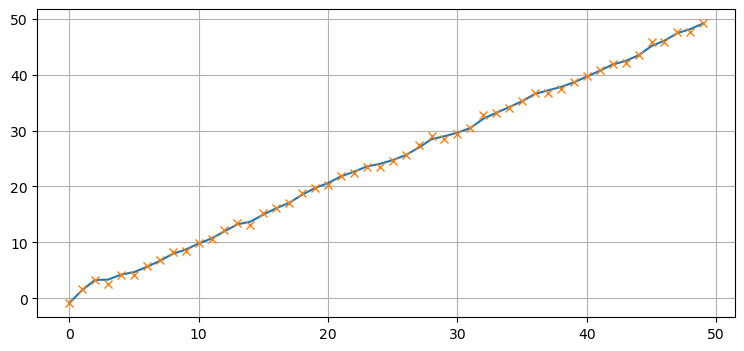There really isn't a lot new here. You have to create an object that creates sigma points for you, and provide functions instead of matrices for $\mathbf F$ and $\mathbf H$, but the rest is the same as before. This should give you enough confidence to plow through a bit of math and algorithms so you can understand what the UKF is doing.

## Choosing Sigma Points¶

At the start of the chapter I used 500,000 randomly generated sigma points to compute the the probability distribution of a Gaussian passed through a nonlinear function. While the computed mean is quite accurate, computing 500,000 points for every update would cause our filter to be extremely slow. So, what would be fewest number of sampled points that we can use, and what kinds of constraints does this problem formulation put on the points? We will assume that we have no special knowledge about the nonlinear function as we want to find a generalized algorithm that works for any function.

Let's consider the simplest possible case and see if it offers any insight. The simplest possible system is the identity function: $f(x) = x$. If our algorithm does not work for the identity function then the filter cannot converge. In other words, if the input is 1 (for a one dimensional system), the output must also be 1. If the output was different, such as 1.1, then when we fed 1.1 into the transform at the next time step, we'd get out yet another number, maybe 1.23. This filter diverges.

The fewest number of points that we can use is one per dimension. This is the number that the linear Kalman filter uses. The input to a Kalman filter for the distribution $\mathcal{N}(\mu,\sigma^2)$ is $\mu$ itself. So while this works for the linear case, it is not a good answer for the nonlinear case.

Perhaps we can use one point per dimension, but altered somehow. However, if we were to pass some value $\mu+\Delta$ into the identity function $f(x)=x$ it would not converge, so this will not work. If we didn't alter $\mu$ then this would be the standard Kalman filter. We must conclude that one sample will not work.

What is the next lowest number we can choose? Two. Consider the fact that Gaussians are symmetric, and that we probably want to always have one of our sample points be the mean of the input for the identity function to work. Two points would require us to select the mean, and then one other point. That one other point would introduce an asymmetry in our input that we probably don't want. It would be very difficult to make this work for the identity function $f(x)=x$.

The next lowest number is 3 points. 3 points allows us to select the mean, and then one point on each side of the mean, as depicted on the chart below.

In :
ukf_internal.show_3_sigma_points()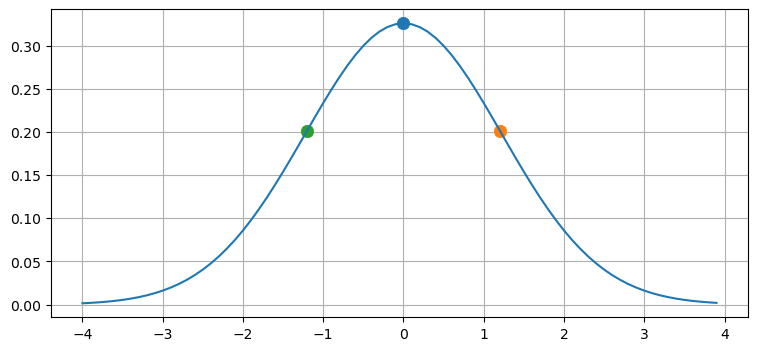We can pass these points through a nonlinear function f(x) and compute the resulting mean and variance. The mean can be computed as the average of the 3 points, but that is not very general. For example, for a very nonlinear problem we might want to weight the center point much higher than the outside points, or we might want to weight the outside points higher.

A more general approach is to compute the weighted mean $\mu = \sum_i w_i\, f(\mathcal{X}_i)$, where the calligraphic $\mathcal{X}$ are the sigma points. We need the sums of the weights to equal one. Given that requirement, our task is to select $\mathcal{X}$ and their corresponding weights so that they compute to the mean and variance of the transformed sigma points.

If we weight the means it also makes sense to weight the covariances. It is possible to use different weights for the mean ($w^m$) and for the covariance ($w^c$). I use superscripts to allow space for indexes in the following equations. We can write

\begin{aligned} \mathbf{Constraints:}\\ 1 &= \sum_i{w_i^m} \\ 1 &= \sum_i{w_i^c} \\ \mu &= \sum_i w_i^mf(\mathcal{X}_i) \\ \Sigma &= \sum_i w_i^c{(f(\mathcal{X})_i-\mu)(f(\mathcal{X})_i-\mu)^\mathsf{T}} \end{aligned}

The first two equations are the constraint that the weights must sum to one. The third equation is how you compute a weight mean. The forth equation may be less familiar, but recall that the equation for the covariance of two random variables is:

$$COV(x,y) = \frac{\sum(x-\bar x)(y-\bar{y})}{n}$$

These constraints do not form a unique solution. For example, if you make $w^m_0$ smaller you can compensate by making $w^m_1$ and $w^m_2$ larger. You can use different weights for the mean and covariances, or the same weights. Indeed, these equations do not require that any of the points be the mean of the input at all, though it seems 'nice' to do so, so to speak.

We want an algorithm that satisfies the constraints, preferably with only 3 points per dimension. Before we go on I want to make sure the idea is clear. Below are three different examples for the same covariance ellipse with different sigma points. The size of the sigma points is proportional to the weight given to each.

In :
ukf_internal.show_sigma_selections()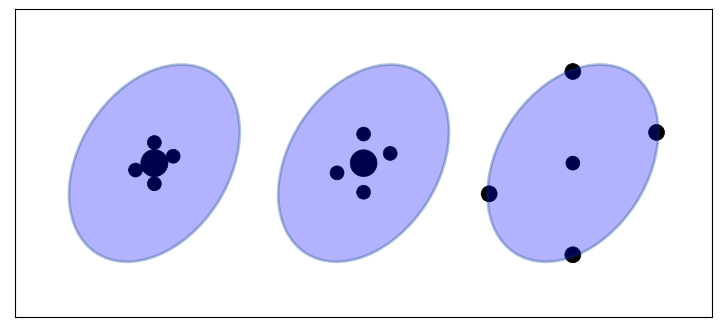The points do not lie along the major and minor axis of the ellipse; nothing in the constraints require me to do that. I show the points evenly spaced, but the constraints do not require that.

The arrangement and weighting of the sigma points affect how we sample the distribution. Points that are close together will sample local effects, and thus probably work better for very nonlinear problems. Points that are far apart, or far off the axis of the ellipse will sample non-local effects and non Gaussian behavior. However, by varying the weights used for each point we can mitigate this. If the points are far from the mean but weighted very slightly we will incorporate some of the knowledge about the distribution without allowing the nonlinearity of the problem to create a bad estimate.

Please understand there are infinite ways to select sigma points. The constraints I choose are just one way to do it. For example, not all algorithms for creating the sigma points require the weights to sum to one. In fact, the algorithm I favor in this book does not have that property.

## The Unscented Transform¶

For the moment, assume an algorithm for selecting the sigma points and weights exists. How are the sigma points used to implement a filter?

The unscented transform is the core of the algorithm yet it is remarkably simple. It passes the sigma points $\boldsymbol{\chi}$ through a nonlinear function yielding a transformed set of points.

$$\boldsymbol{\mathcal{Y}} = f(\boldsymbol{\chi})$$

It then computes the mean and covariance of the transformed points. That mean and covariance becomes the new estimate. The figure below depicts the operation of the unscented transform. The green ellipse on the right represents the computed mean and covariance to the transformed sigma points.

In :
ukf_internal.show_sigma_transform(with_text=True)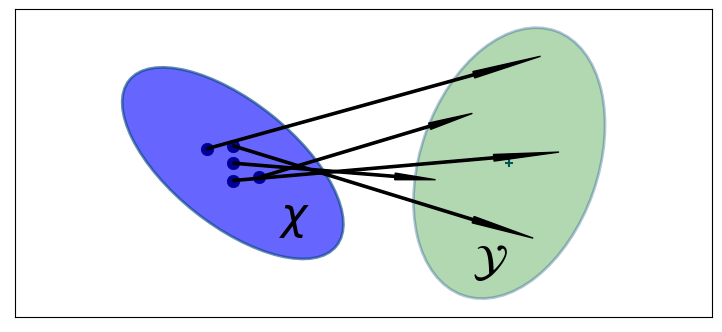The mean and covariance of the sigma points are computed as:

\begin{aligned} \mu &= \sum_{i=0}^{2n} w^m_i\boldsymbol{\mathcal{Y}}_i \\ \Sigma &= \sum_{i=0}^{2n} w^c_i{(\boldsymbol{\mathcal{Y}}_i-\mu)(\boldsymbol{\mathcal{Y}}_i-\mu)^\mathsf{T}} \end{aligned}

These equations should be familiar - they are the constraint equations we developed above.

In short, the unscented transform takes points sampled from some arbitary probability distribution, passes them through an arbitrary, nonlinear function and produces a Gaussian for each transformed points. I hope you can envision how we can use this to implement a nonlinear Kalman filter. Once we have Gaussians all of the mathematical apparatus we have already developed comes into play!

The name "unscented" might be confusing. It doesn't really mean much. It was a joke fostered by the inventor that his algorithm didn't "stink", and soon the name stuck. There is no mathematical meaning to the term.

### Accuracy of the Unscented Transform¶

Earlier we wrote a function that found the mean of a distribution by passing 50,000 points through a nonlinear function. Let's now pass 5 sigma points through the same function, and compute their mean with the unscented transform. We will use the FilterPy function MerweScaledSigmaPoints() to create the sigma points and unscented_transform to perform the transform; we will learn about these functions later. In the first example in this chapter I used JulierSigmaPoints; they both choose sigma points, but in different ways which I will explain later.

In :
from filterpy.kalman import unscented_transform, MerweScaledSigmaPoints
import scipy.stats as stats

#initial mean and covariance
mean = (0., 0.)
p = np.array([[32., 15], [15., 40.]])

# create sigma points and weights
points = MerweScaledSigmaPoints(n=2, alpha=.3, beta=2., kappa=.1)
sigmas = points.sigma_points(mean, p)

### pass through nonlinear function
sigmas_f = np.empty((5, 2))
for i in range(5):
sigmas_f[i] = f_nonlinear_xy(sigmas[i, 0], sigmas[i ,1])

### use unscented transform to get new mean and covariance
ukf_mean, ukf_cov = unscented_transform(sigmas_f, points.Wm, points.Wc)

#generate random points
np.random.seed(100)
xs, ys = multivariate_normal(mean=mean, cov=p, size=5000).T

plot_monte_carlo_mean(xs, ys, f_nonlinear_xy, ukf_mean, 'Unscented Mean')
ax = plt.gcf().axes
ax.scatter(sigmas[:,0], sigmas[:,1], c='r', s=30);

Difference in mean x=-0.097, y=0.549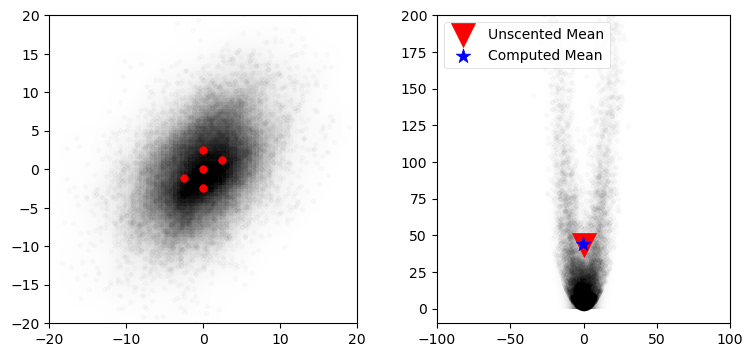I find this result remarkable. Using only 5 points we were able to compute the mean with amazing accuracy. The error in x is only -0.097, and the error in y is 0.549. In contrast, a linearized approach (used by the EKF, which we will learn in the next chapter) gave an error of over 43 in y. If you look at the code that generates the sigma points you'll see that it has no knowledge of the nonlinear function, only of the mean and covariance of our initial distribution. The same 5 sigma points would be generated if we had a completely different nonlinear function.

I will admit to choosing a nonlinear function that makes the performance of the unscented tranform striking compared to the EKF. But the physical world is filled with very nonlinear behavior, and the UKF takes it in stride. I did not 'work' to find a function where the unscented transform happened to work well. You will see in the next chapter how more traditional techniques struggle with strong nonlinearities. This graph is the foundation of why I advise you to use the UKF or similar modern technique whenever possible.

## The Unscented Kalman Filter¶

We can now present the UKF algorithm.

### Predict Step¶

The UKF's predict step computes the prior using the process model $f()$. $f()$ is assumed to be nonlinear, so we generate sigma points $\mathcal{X}$ and their corresponding weights $W^m, W^c$ according to some function:

\begin{aligned} \boldsymbol\chi &= \text{sigma-function}(\mathbf x, \mathbf P) \\ W^m, W^c &= \text{weight-function}(\mathtt{n, parameters})\end{aligned}

We pass each sigma point through $f(\mathbf x, \Delta t)$. This projects the sigma points forward in time according to the process model, forming the new prior, which is a set of sigma points we name $\boldsymbol{\mathcal Y}$:

$$\boldsymbol{\mathcal{Y}} = f(\boldsymbol{\chi}, \Delta t)$$

We compute the mean and covariance of the prior using the unscented transform on the transformed sigma points.

$$\mathbf{\bar x}, \mathbf{\bar P} = UT(\mathcal{Y}, w_m, w_c, \mathbf Q)$$

These are the equations for the unscented transform:

\begin{aligned} \mathbf{\bar x} &= \sum_{i=0}^{2n} w^m_i\boldsymbol{\mathcal Y}_i \\ \mathbf{\bar P} &= \sum_{i=0}^{2n} w^c_i({\boldsymbol{\mathcal Y}_i - \mathbf{\bar x})(\boldsymbol{\mathcal Y}_i-\mathbf{\bar x})^\mathsf{T}} + \mathbf Q \end{aligned}

This table compares the linear Kalman filter with the Unscented Kalman Filter equations. I've dropped the subscript $i$ for readability.

$$\begin{array}{l|l} \text{Kalman} & \text{Unscented} \\ \hline & \boldsymbol{\mathcal Y} = f(\boldsymbol\chi) \\ \mathbf{\bar x} = \mathbf{Fx} & \mathbf{\bar x} = \sum w^m\boldsymbol{\mathcal Y} \\ \mathbf{\bar P} = \mathbf{FPF}^\mathsf T + \mathbf Q & \mathbf{\bar P} = \sum w^c({\boldsymbol{\mathcal Y} - \mathbf{\bar x})(\boldsymbol{\mathcal Y} - \mathbf{\bar x})^\mathsf T}+\mathbf Q \end{array}$$

### Update Step¶

Kalman filters perform the update in measurement space. Thus we must convert the sigma points of the prior into measurements using a measurement function $h(x)$ that you define.

$$\boldsymbol{\mathcal{Z}} = h(\boldsymbol{\mathcal{Y}})$$

We compute the mean and covariance of these points using the unscented transform. The $z$ subscript denotes that these are the mean and covariance of the measurement sigma points.

\begin{aligned} \boldsymbol\mu_z, \mathbf P_z &= UT(\boldsymbol{\mathcal Z}, w_m, w_c, \mathbf R) \\ \boldsymbol\mu_z &= \sum_{i=0}^{2n} w^m_i\boldsymbol{\mathcal Z}_i \\ \mathbf P_z &= \sum_{i=0}^{2n} w^c_i{(\boldsymbol{\mathcal Z}_i-\boldsymbol{\mu}_z)(\boldsymbol{\mathcal Z}_i-\boldsymbol{\mu}_z)^\mathsf T} + \mathbf R \end{aligned}

Next we compute the residual and Kalman gain. The residual of the measurement $\mathbf z$ is trivial to compute:

$$\mathbf y = \mathbf z - \boldsymbol\mu_z$$

To compute the Kalman gain we first compute the cross covariance of the state and the measurements, which is defined as:

$$\mathbf P_{xz} =\sum_{i=0}^{2n} w^c_i(\boldsymbol{\mathcal Y}_i-\mathbf{\bar x})(\boldsymbol{\mathcal Z}_i-\boldsymbol\mu_z)^\mathsf T$$

And then the Kalman gain is defined as

$$\mathbf{K} = \mathbf P_{xz} \mathbf P_z^{-1}$$

If you think of the inverse as a kind of matrix reciprocal, you can see that the Kalman gain is a simple ratio which computes:

$$\mathbf{K} \approx \frac{\mathbf P_{xz}}{\mathbf P_z} \approx \frac{\text{belief in state}}{\text{belief in measurement}}$$

Finally, we compute the new state estimate using the residual and Kalman gain:

$$\mathbf x = \bar{\mathbf x} + \mathbf{Ky}$$

and the new covariance is computed as:

$$\mathbf P = \mathbf{\bar P} - \mathbf{KP_z}\mathbf{K}^\mathsf{T}$$

This step contains a few equations you have to take on faith, but you should be able to see how they relate to the linear Kalman filter equations. The linear algebra is slightly different from the linear Kalman filter, but the algorithm is the same Bayesian algorithm we have been implementing throughout the book.

This table compares the equations of the linear KF and UKF equations.

$$\begin{array}{l|l} \textrm{Kalman Filter} & \textrm{Unscented Kalman Filter} \\ \hline & \boldsymbol{\mathcal Y} = f(\boldsymbol\chi) \\ \mathbf{\bar x} = \mathbf{Fx} & \mathbf{\bar x} = \sum w^m\boldsymbol{\mathcal Y} \\ \mathbf{\bar P} = \mathbf{FPF}^\mathsf T+\mathbf Q & \mathbf{\bar P} = \sum w^c({\boldsymbol{\mathcal Y} - \mathbf{\bar x})(\boldsymbol{\mathcal Y} - \mathbf{\bar x})^\mathsf T}+\mathbf Q \\ \hline & \boldsymbol{\mathcal Z} = h(\boldsymbol{\mathcal{Y}}) \\ & \boldsymbol\mu_z = \sum w^m\boldsymbol{\mathcal{Z}} \\ \mathbf y = \mathbf z - \mathbf{Hx} & \mathbf y = \mathbf z - \boldsymbol\mu_z \\ \mathbf S = \mathbf{H\bar PH}^\mathsf{T} + \mathbf R & \mathbf P_z = \sum w^c{(\boldsymbol{\mathcal Z}-\boldsymbol\mu_z)(\boldsymbol{\mathcal{Z}}-\boldsymbol\mu_z)^\mathsf{T}} + \mathbf R \\ \mathbf K = \mathbf{\bar PH}^\mathsf T \mathbf S^{-1} & \mathbf K = \left[\sum w^c(\boldsymbol{\mathcal Y}-\bar{\mathbf x})(\boldsymbol{\mathcal{Z}}-\boldsymbol\mu_z)^\mathsf{T}\right] \mathbf P_z^{-1} \\ \mathbf x = \mathbf{\bar x} + \mathbf{Ky} & \mathbf x = \mathbf{\bar x} + \mathbf{Ky}\\ \mathbf P = (\mathbf{I}-\mathbf{KH})\mathbf{\bar P} & \mathbf P = \bar{\mathbf P} - \mathbf{KP_z}\mathbf{K}^\mathsf{T} \end{array}$$

## Van der Merwe's Scaled Sigma Point Algorithm¶

There are many algorithms for selecting sigma points. Since 2005 or so research and industry have mostly settled on the version published by Rudolph Van der Merwe in his 2004 PhD dissertation . It performs well with a variety of problems and it has a good tradeoff between performance and accuracy. It is a slight reformulation of the Scaled Unscented Transform published by Simon J. Julier .

This formulation uses 3 parameters to control how the sigma points are distributed and weighted: $\alpha$, $\beta$, and $\kappa$. Before we work through the equations, let's look at an example. I will plot the sigma points on top of a covariance ellipse showing the first and second standard deviations, and scale the points based on the mean weights.

In :
ukf_internal.plot_sigma_points()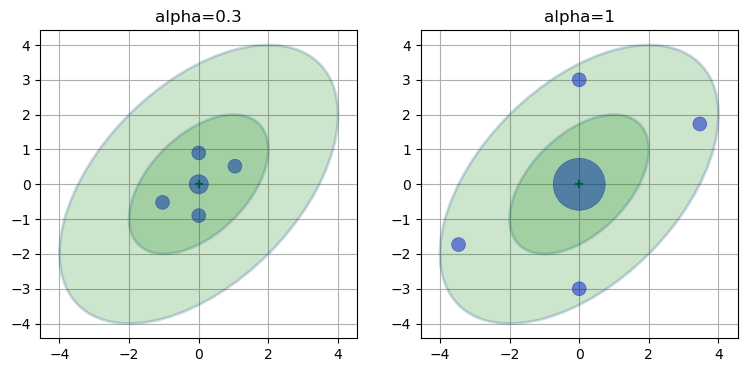We can see that the sigma points lie between the first and second standard deviation, and that the larger $\alpha$ spreads the points out. Furthermore, the larger $\alpha$ weights the mean (center point) higher than the smaller $\alpha$, and weights the rest less. This should fit our intuition - the further a point is from the mean the less we should weight it. We don't know how these weights and sigma points are selected yet, but the choices look reasonable.

### Sigma Point Computation¶

The first sigma point is the mean of the input. This is the sigma point displayed in the center of the ellipses in the diagram above. We will call this $\boldsymbol{\chi}_0$.

$$\mathcal{X}_0 = \mu$$

For notational convenience we define $\lambda = \alpha^2(n+\kappa)-n$, where $n$ is the dimension of $\mathbf x$. The remaining sigma points are computed as

$$\boldsymbol{\chi}_i = \begin{cases} \mu + \left[ \sqrt{(n+\lambda)\Sigma}\right ]_{i}& i=1..n \\ \mu - \left[ \sqrt{(n+\lambda)\Sigma}\right]_{i-n} &i=(n+1)..2n\end{cases}$$

The $i$ subscript chooses the i$^{th}$ row vector of the matrix.

In other words, we scale the covariance matrix by a constant, take the square root of it, and ensure symmetry by both adding and subtracting it from the mean. We will discuss how you take the square root of a matrix later.

### Weight Computation¶

This formulation uses one set of weights for the means, and another set for the covariance. The weights for the mean of $\mathcal{X}_0$ is computed as

$$W^m_0 = \frac{\lambda}{n+\lambda}$$

The weight for the covariance of $\mathcal{X}_0$ is

$$W^c_0 = \frac{\lambda}{n+\lambda} + 1 -\alpha^2 + \beta$$

The weights for the rest of the sigma points $\boldsymbol{\chi}_1 ... \boldsymbol{\chi}_{2n}$ are the same for the mean and covariance. They are

$$W^m_i = W^c_i = \frac{1}{2(n+\lambda)}\;\;\;i=1..2n$$

It may not be obvious why this is 'correct', and indeed, it cannot be proven that this is ideal for all nonlinear problems. But you can see that we are choosing the sigma points proportional to the square root of the covariance matrix, and the square root of variance is standard deviation. So, the sigma points are spread roughly according to $\pm 1\sigma$ times some scaling factor. There is an $n$ term in the denominator, so with more dimensions the points will be spread out and weighed less.

Important note: Ordinarily these weights do not sum to one. I get many questions about this. Getting weights that sum to greater than one, or even negative values is expected. I cover this in more detail below.

### Reasonable Choices for the Parameters¶

$\beta=2$ is a good choice for Gaussian problems, $\kappa=3-n$ where $n$ is the dimension of $\mathbf x$ is a good choice for $\kappa$, and $0 \le \alpha \le 1$ is an appropriate choice for $\alpha$, where a larger value for $\alpha$ spreads the sigma points further from the mean.

## Using the UKF¶

Let's solve some problems so you can gain confidence in how easy the UKF is to use. We will start with a linear problem you already know how to solve with the linear Kalman filter. Although the UKF was designed for nonlinear problems, it finds the same optimal result as the linear Kalman filter for linear problems. We will write a filter to track an object in 2D using a constant velocity model. This will allow us to focus on what is the same (and most is the same!) and what is different with the UKF.

Designing a Kalman filter requires you to specify the $\bf{x}$, $\bf{F}$, $\bf{H}$, $\bf{R}$, and $\bf{Q}$ matrices. We have done this many times so I will give you the matrices without a lot of discussion. We want a constant velocity model, so we define $\bf{x}$ to be

$$\mathbf x = \begin{bmatrix}x & \dot x & y & \dot y \end{bmatrix}^\mathsf{T}$$

With this ordering of state variables the state transition matrix is

$$\mathbf F = \begin{bmatrix}1 & \Delta t & 0 & 0 \\ 0&1&0&0 \\ 0&0&1&\Delta t\\ 0&0&0&1 \end{bmatrix}$$

which implement the Newtonian equations

\begin{aligned} x_k &= x_{k-1} + \dot x_{k-1}\Delta t \\ y_k &= y_{k-1} + \dot y_{k-1}\Delta t \end{aligned}

Our sensors provide position but not velocity, so the measurement function is

$$\mathbf H = \begin{bmatrix}1&0&0&0 \\ 0&0&1&0 \end{bmatrix}$$

The sensor readings are in meters with an error of $\sigma=0.3$ meters in both x and y. This gives us a measurement noise matrix of

$$\mathbf R = \begin{bmatrix}0.3^2 &0\\0 & 0.3^2\end{bmatrix}$$

Finally, let's assume that the process noise can be represented by the discrete white noise model - that is, that over each time period the acceleration is constant. We can use FilterPy's Q_discrete_white_noise() to create this matrix for us, but for review the matrix is

$$\mathbf Q = \begin{bmatrix} \frac{1}{4}\Delta t^4 & \frac{1}{2}\Delta t^3 \\ \frac{1}{2}\Delta t^3 & \Delta t^2\end{bmatrix} \sigma^2$$

My implementation of this filter is:

In :
from filterpy.kalman import KalmanFilter
from filterpy.common import Q_discrete_white_noise
from numpy.random import randn

std_x, std_y = .3, .3
dt = 1.0

np.random.seed(1234)
kf = KalmanFilter(4, 2)
kf.x = np.array([0., 0., 0., 0.])
kf.R = np.diag([std_x**2, std_y**2])
kf.F = np.array([[1, dt, 0, 0],
[0, 1, 0, 0],
[0, 0, 1, dt],
[0, 0, 0, 1]])
kf.H = np.array([[1, 0, 0, 0],
[0, 0, 1, 0]])

kf.Q[0:2, 0:2] = Q_discrete_white_noise(2, dt=1, var=0.02)
kf.Q[2:4, 2:4] = Q_discrete_white_noise(2, dt=1, var=0.02)

zs = [np.array([i + randn()*std_x,
i + randn()*std_y]) for i in range(100)]
xs, _, _, _ = kf.batch_filter(zs)
plt.plot(xs[:, 0], xs[:, 2]);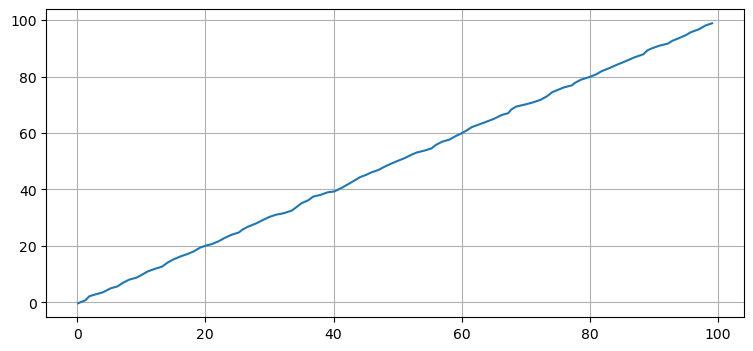This should hold no surprises for you. Now let's implement a UKF. Again, this is purely for educational purposes; using a UKF for a linear problem confers no benefit. FilterPy implements the UKF with the class UnscentedKalmanFilter.

The first thing to do is implement the functions f(x, dt) and h(x). f(x, dt) implements the state transition function, and h(x) implements the measurement function. These correspond to the matrices $\mathbf F$ and $\mathbf H$ in the linear filter.

Below is a reasonable implementation of these two functions. Each is expected to return a 1D NumPy array or list containing the result. You may give them more readable names than f and h.

In :
def f_cv(x, dt):
""" state transition function for a
constant velocity aircraft"""

F = np.array([[1, dt, 0,  0],
[0,  1, 0,  0],
[0,  0, 1, dt],
[0,  0, 0,  1]])
return F @ x

def h_cv(x):
return x[[0, 2]]


Next you specify how to compute the sigma points and weights. We gave Van der Merwe's version above, but there are many different choices. FilterPy uses a class named SigmaPoints which must implement a method:

def sigma_points(self, x, P)


and contain attributes Wm and Wc, which hold the weights for computing the mean and covariance, respectively.

FilterPy derives the class MerweScaledSigmaPoints from SigmaPoints and implements the aforementioned methods.

When you create the UKF you will pass in the $f()$ and $h()$ functions and the sigma point object, as in this example:

from filterpy.kalman import MerweScaledSigmaPoints
from filterpy.kalman import UnscentedKalmanFilter as UKF

points = MerweScaledSigmaPoints(n=4, alpha=.1, beta=2., kappa=-1)
ukf = UKF(dim_x=4, dim_z=2, fx=f_cv, hx=h_cv, dt=dt, points=points)


The rest of the code is the same as for the linear kalman filter. I'll use the same measurements and compute the standard deviation of the difference between the two solutions.

In :
from filterpy.kalman import UnscentedKalmanFilter as UKF

import numpy as np

sigmas = MerweScaledSigmaPoints(4, alpha=.1, beta=2., kappa=1.)
ukf = UKF(dim_x=4, dim_z=2, fx=f_cv,
hx=h_cv, dt=dt, points=sigmas)
ukf.x = np.array([0., 0., 0., 0.])
ukf.R = np.diag([0.09, 0.09])
ukf.Q[0:2, 0:2] = Q_discrete_white_noise(2, dt=1, var=0.02)
ukf.Q[2:4, 2:4] = Q_discrete_white_noise(2, dt=1, var=0.02)

uxs = []
for z in zs:
ukf.predict()
ukf.update(z)
uxs.append(ukf.x.copy())
uxs = np.array(uxs)

plt.plot(uxs[:, 0], uxs[:, 2])
print(f'UKF standard deviation {np.std(uxs - xs):.3f} meters')

UKF standard deviation 0.013 meters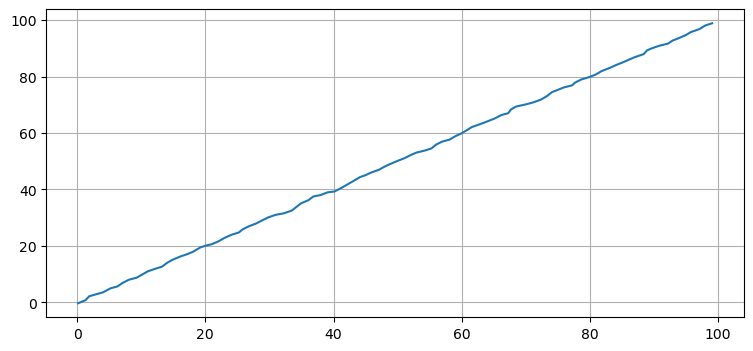This gave me a standard deviation of 0.013 meters, which is quite small.

The implementation of the UKF is not that different from the linear Kalman filter. Instead of implementing the state transition and measurement functions as the matrices $\mathbf F$ and $\mathbf H$ you supply nonlinear functions f() and h(). The rest of the theory and implementation remains the same. The code implementing predict() and update() differs, but from a designer's point of view the problem formulation and filter design is very similar.

## Tracking an Airplane¶

Let's tackle our first nonlinear problem. We will write a filter to track an airplane using radar as the sensor. To keep the problem as similar to the previous one as possible we will track in two dimensions. We will track one dimension on the ground and the altitude of the aircraft. Each dimension is independent so we can do this with no loss of generality.

Radars work by emitting radio waves or microwaves. Anything in the beam's path will reflect some of the signal back to the radar. By timing how long it takes for the reflected signal to return it can compute the slant distance to the target. Slant distance is the straight line distance from the radar to the object. Bearing is computed using the directive gain of the antenna.

We compute the (x,y) position of the aircraft from the slant distance and elevation angle as illustrated by this diagram:

In :
import kf_book.ekf_internal as ekf_internal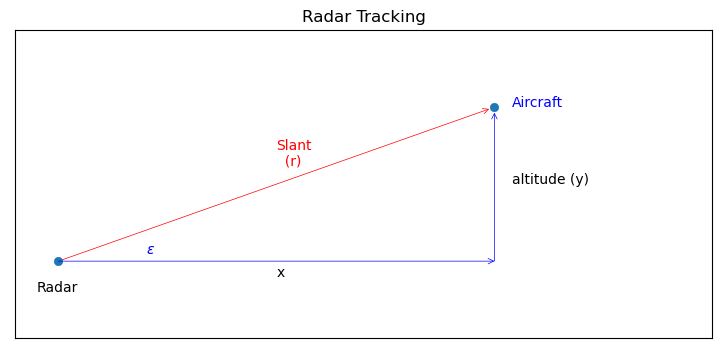The elevation angle $\epsilon$ is the angle above the line of sight formed by the ground.

We will assume that the aircraft is flying at a constant altitude. Thus we have a three variable state vector:

$$\mathbf x = \begin{bmatrix}\mathtt{distance} \\\mathtt{velocity}\\ \mathtt{altitude}\end{bmatrix}= \begin{bmatrix}x \\ \dot x\\ y\end{bmatrix}$$

The state transition function is linear

$$\mathbf{\bar x} = \begin{bmatrix} 1 & \Delta t & 0 \\ 0& 1& 0 \\ 0&0&1\end{bmatrix} \begin{bmatrix}x \\ \dot x\\ y\end{bmatrix}$$

and can be computed with:

In :
def f_radar(x, dt):
""" state transition function for a constant velocity
aircraft with state vector [x, velocity, altitude]'"""

F = np.array([[1, dt, 0],
[0,  1, 0],
[0,  0, 1]], dtype=float)
return F @ x


Next we design the measurement function. As in the linear Kalman filter the measurement function converts the filter's prior into a measurement. We need to convert the position and velocity of the aircraft into the elevation angle and range from the radar station.

Range is computed with the Pythagorean theorem:

$$\text{range} = \sqrt{(x_\text{ac} - x_\text{radar})^2 + (y_\text{ac} - y_\mathtt{radar})^2}$$

The elevation angle $\epsilon$ is the arctangent of $y/x$:

$$\epsilon = \tan^{-1}{\frac{y_\mathtt{ac} - y_\text{radar}}{x_\text{ac} - x_\text{radar}}}$$

We need to define a Python function to compute this. I'll take advantage of the fact that a function can own a variable to store the radar's position. While this isn't necessary for this problem (we could hard code the value, or use a global), this gives the function more flexibility.

In :
def h_radar(x):
slant_range = math.sqrt(dx**2 + dy**2)
elevation_angle = math.atan2(dy, dx)
return [slant_range, elevation_angle]



There is a nonlinearity that we are not considering, the fact that angles are modular. The residual is the difference between the measurement and the prior projected into measurement space. The angular difference between 359° and 1° is 2°, but 359° - 1° = 358°. This is exacerbated by the UKF which computes sums of weighted values in the unscented transform. For now we will place our sensors and targets in positions that avoid these nonlinear regions. Later I will show you how to handle this problem.

We need to simulate the radar and the aircraft. By now this should be second nature for you, so I offer the code without discussion.

In :
from numpy.linalg import norm
from math import atan2

def __init__(self, pos, range_std, elev_angle_std):
self.pos = np.asarray(pos)
self.range_std = range_std
self.elev_angle_std = elev_angle_std

""" Returns (range, elevation angle) to aircraft.
"""

diff = np.subtract(ac_pos, self.pos)
rng = norm(diff)
brg = atan2(diff, diff)
return rng, brg

""" Compute range and elevation angle to aircraft with
simulated noise"""

rng += randn() * self.range_std
brg += randn() * self.elev_angle_std
return rng, brg

class ACSim:
def __init__(self, pos, vel, vel_std):
self.pos = np.asarray(pos, dtype=float)
self.vel = np.asarray(vel, dtype=float)
self.vel_std = vel_std

def update(self, dt):
""" Compute and returns next position. Incorporates
random variation in velocity. """

dx = self.vel*dt + (randn() * self.vel_std) * dt
self.pos += dx
return self.pos


A military grade radar achieves 1 meter RMS range accuracy, and 1 mrad RMS for elevation angle . We will assume a more modest 5 meter range accuracy, and 0.5° angular accuracy as this provides a more challenging data set for the filter.

The design of $\mathbf Q$ requires some discussion. The state is $\begin{bmatrix}x & \dot x & y\end{bmatrix}^\mathtt{T}$. The first two elements are down range distance and velocity, so we can use Q_discrete_white_noise noise to compute the values for the upper left hand side of Q. The third element is altitude, which we assume is independent of $x$. That results in a block design for $\mathbf Q$:

$$\mathbf Q = \begin{bmatrix}\mathbf Q_\mathtt{x} & \boldsymbol 0 \\ \boldsymbol 0 & Q_\mathtt{y}\end{bmatrix}$$

I'll start with the aircraft positioned directly over the radar station, flying at 100 m/s. A typical height finder radar might update only once every 3 seconds so we will use that for our epoch period.

In :
import math

dt = 3. # 12 seconds between readings
range_std = 5 # meters
ac_pos = (0., 1000.)
ac_vel = (100., 0.)

points = MerweScaledSigmaPoints(n=3, alpha=.1, beta=2., kappa=0.)

kf.Q[0:2, 0:2] = Q_discrete_white_noise(2, dt=dt, var=0.1)
kf.Q[2,2] = 0.1

kf.R = np.diag([range_std**2, elevation_angle_std**2])
kf.x = np.array([0., 90., 1100.])
kf.P = np.diag([300**2, 30**2, 150**2])

np.random.seed(200)
pos = (0, 0)